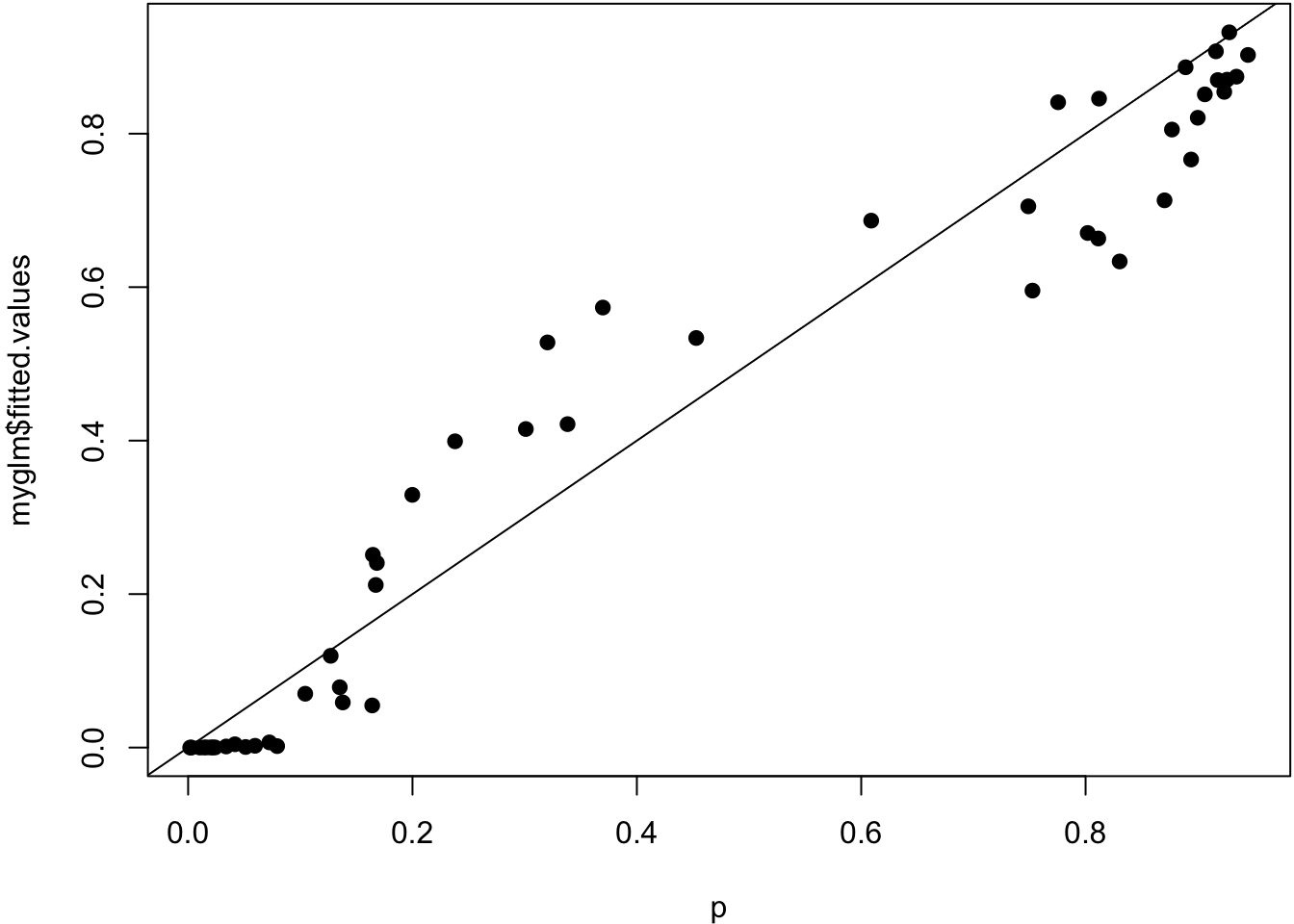68.1 Ordinary Least Squares

Recall that OLS estimates the model

\begin{aligned} Y_i & = \beta_1 X_{i1} + \beta_2 X_{i2} + \ldots + \beta_p X_{ip} + E_i \\ & = {\boldsymbol{X}}_i {\boldsymbol{\beta}}+ E_i \end{aligned}

where $${\operatorname{E}}[{\boldsymbol{E}}| {\boldsymbol{X}}] = {\boldsymbol{0}}$$ and $${\operatorname{Cov}}({\boldsymbol{E}}| {\boldsymbol{X}}) = \sigma^2 {\boldsymbol{I}}$$.

The additive model (which could also be called “ordinary nonparametric additive regression”) is of the form

\begin{aligned} Y_i & = s_1(X_{i1}) + s_2(X_{i2}) + \ldots + s_p(X_{ip}) + E_i \\ & = \sum_{j=1}^p s_j(X_{ij}) + E_i \end{aligned}

where the $$s_j(\cdot)$$ for $$j = 1, \ldots, p$$ are a set of nonparametric (or flexible) functions. Again, we assume that $${\operatorname{E}}[{\boldsymbol{E}}| {\boldsymbol{X}}] = {\boldsymbol{0}}$$ and $${\operatorname{Cov}}({\boldsymbol{E}}| {\boldsymbol{X}}) = \sigma^2 {\boldsymbol{I}}$$.

68.3 Backfitting

The additive model can be fit through a technique called backfitting.

1. Intialize $$s^{(0)}_j(x)$$ for $$j = 1, \ldots, p$$.
2. For $$t=1, 2, \ldots$$, fit $$s_j^{(t)}(x)$$ on response variable $y_i - \sum_{k \not= j} s_k^{(t-1)}(x_{ij}).$
3. Repeat until convergence.

Note that some extra steps have to be taken to deal with the intercept.

68.4 GAM Definition

$$Y | {\boldsymbol{X}}$$ is distributed according to an exponential family distribution. The extension of additive models to this family of response variable is called generalized additive models (GAMs). The model is of the form

$g\left({\operatorname{E}}[Y_i | {\boldsymbol{X}}_i]\right) = \sum_{j=1}^p s_j(X_{ij})$

where $$g(\cdot)$$ is the link function and the $$s_j(\cdot)$$ are flexible and/or nonparametric functions.

68.5 Overview of Fitting GAMs

Fitting GAMs involves putting together the following three tools:

1. We know how to fit a GLM via IRLS
2. We know how to fit a smoother of a single explanatory variable via a least squares solution, as seen for the NCS
3. We know how to combine additive smoothers by backfitting

68.6 GAMs in R

Three common ways to fit GAMs in R:

1. Utilize glm() on explanatory variables constructed from ns() or bs()
2. The gam library
3. The mgcv library

68.7 Example

> set.seed(508)
> x1 <- seq(1, 10, length.out=50)
> n <- length(x1)
> x2 <- rnorm(n)
> f <- 4*log(x1) + sin(x1) - 7 + 0.5*x2
> p <- exp(f)/(1+exp(f))
> summary(p)
Min.  1st Qu.   Median     Mean  3rd Qu.     Max.
0.001842 0.074171 0.310674 0.436162 0.860387 0.944761
> y <- rbinom(n, size=1, prob=p)
> mean(y)
 0.42
> df <- data.frame(x1=x1, x2=x2, y=y)

Here, we use the gam() function from the mgcv library. It automates choosing the smoothness of the splines.

> library(mgcv)
> mygam <- gam(y ~ s(x1) + s(x2), family = binomial(), data=df)
> library(broom)
> tidy(mygam)
# A tibble: 2 x 5
term    edf ref.df statistic p.value
<chr> <dbl>  <dbl>     <dbl>   <dbl>
1 s(x1)  1.87   2.37     12.7  0.00531
2 s(x2)  1.00   1.00      1.16 0.281
> summary(mygam)

Family: binomial

Formula:
y ~ s(x1) + s(x2)

Parametric coefficients:
Estimate Std. Error z value Pr(>|z|)
(Intercept)  -1.1380     0.6723  -1.693   0.0905 .
---
Signif. codes:  0 '***' 0.001 '**' 0.01 '*' 0.05 '.' 0.1 ' ' 1

Approximate significance of smooth terms:
edf Ref.df Chi.sq p-value
s(x1) 1.87  2.375 12.743 0.00531 **
s(x2) 1.00  1.000  1.163 0.28084
---
Signif. codes:  0 '***' 0.001 '**' 0.01 '*' 0.05 '.' 0.1 ' ' 1

R-sq.(adj) =  0.488   Deviance explained =   47%
UBRE = -0.12392  Scale est. = 1         n = 50

True probabilities vs. estimated probabilities.

> plot(p, mygam$fitted.values, pch=19); abline(0,1)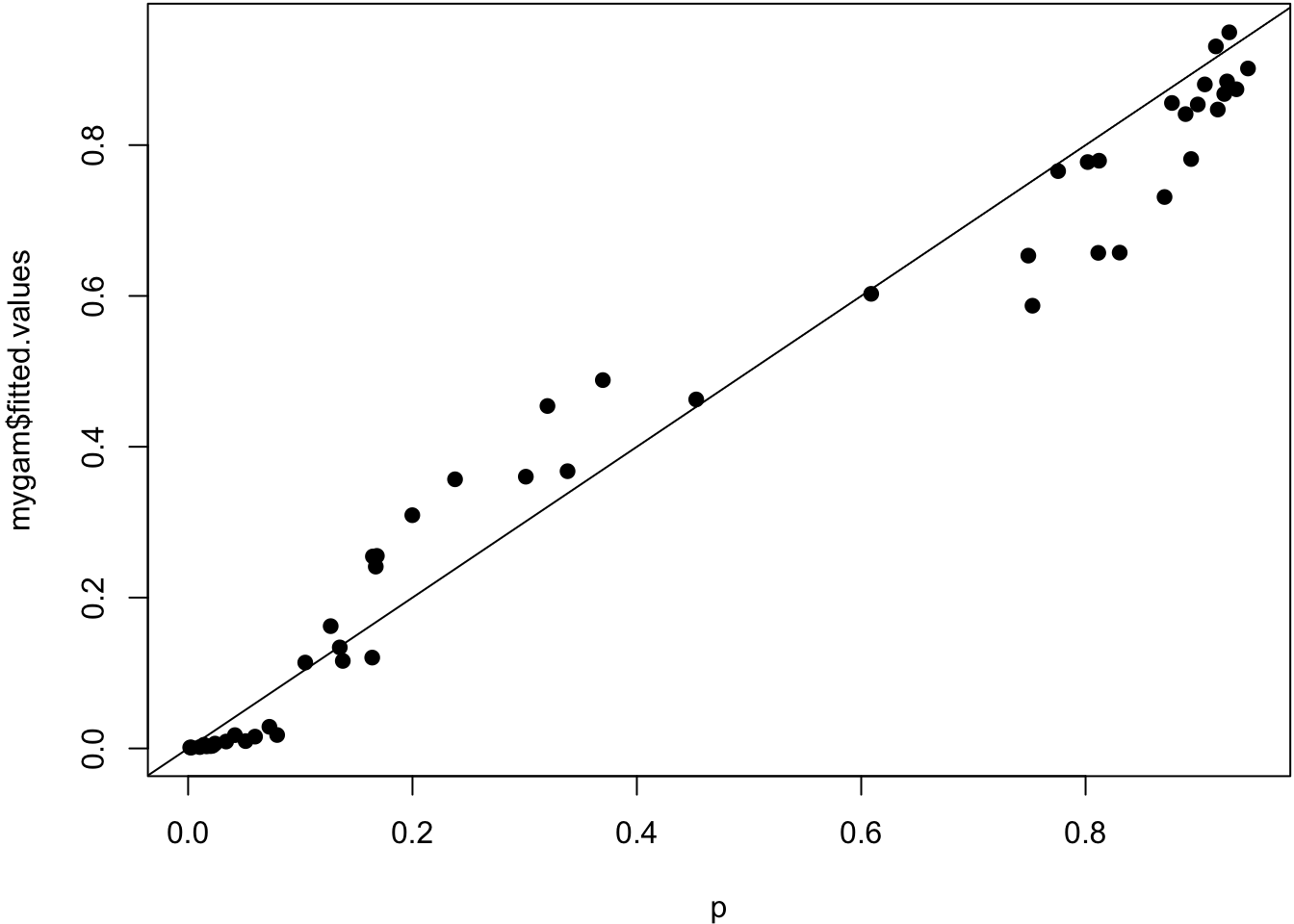Smoother estimated for x1. > plot(mygam, select=1)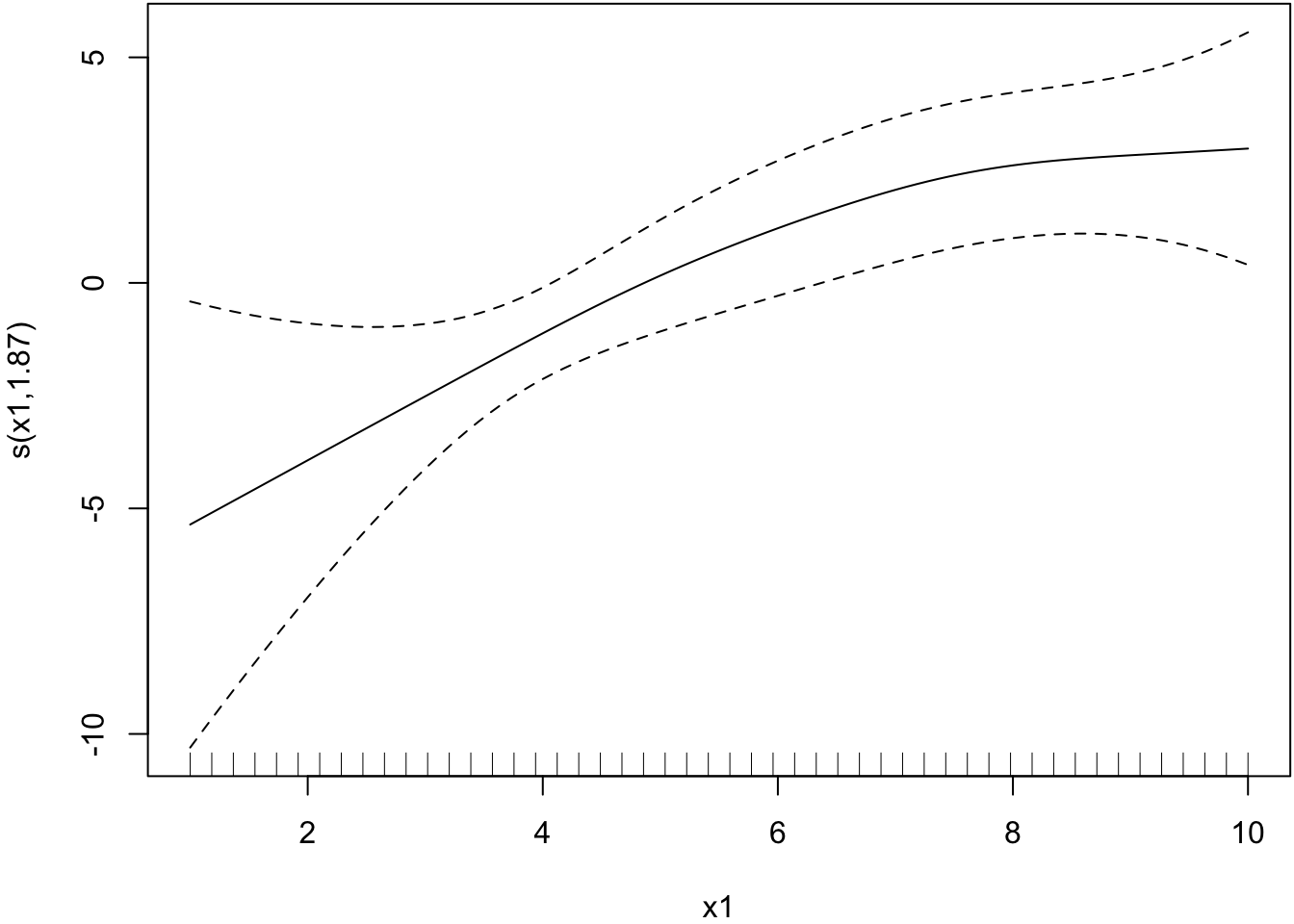Smoother estimated for x2. > plot(mygam, select=2)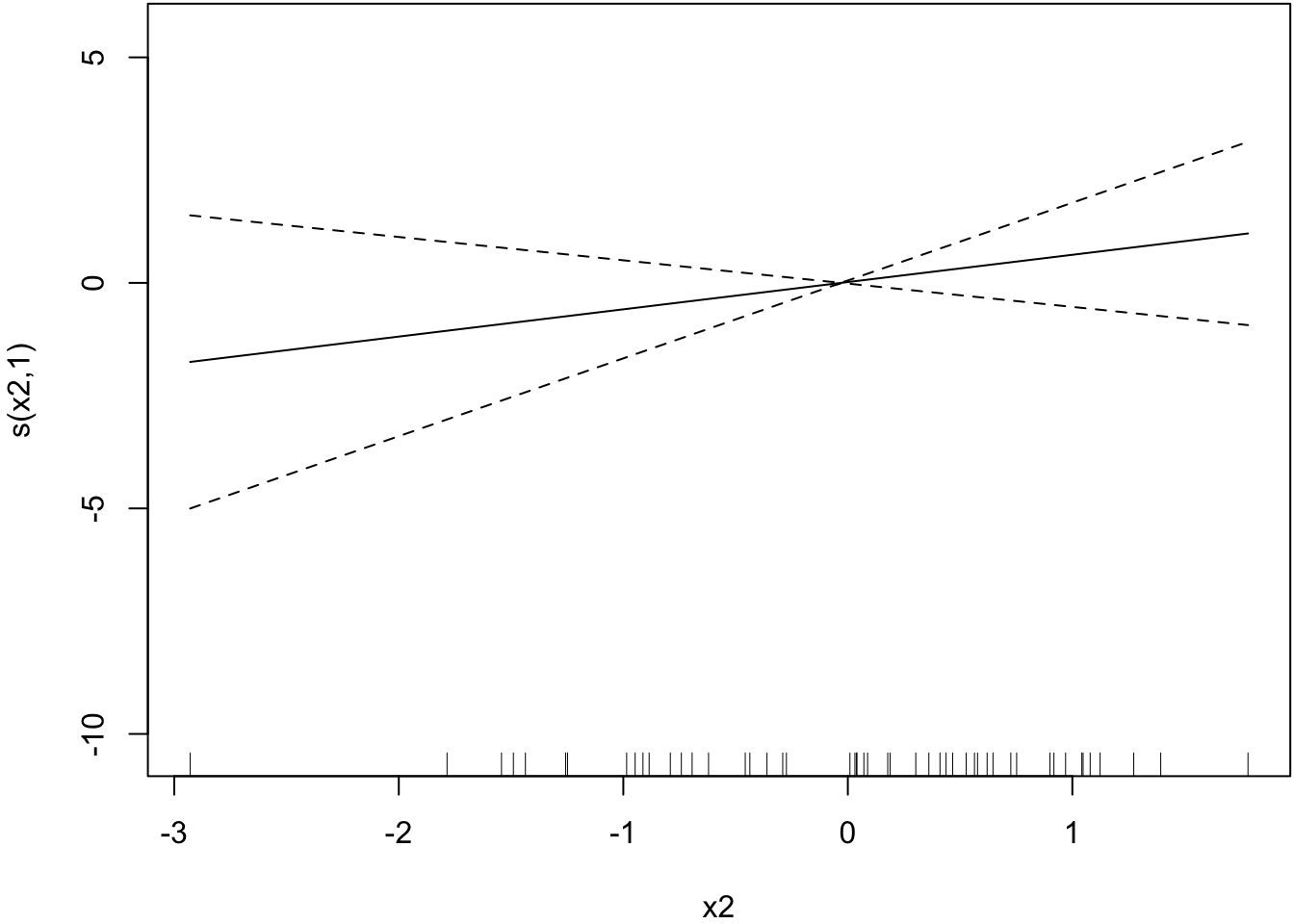Here, we use the glm() function and include as an explanatory variable a NCS built from the ns() function from the splines library. We include a df argument in the ns() call. > library(splines) > myglm <- glm(y ~ ns(x1, df=2) + x2, family = binomial(), data=df) > tidy(myglm) # A tibble: 4 x 5 term estimate std.error statistic p.value <chr> <dbl> <dbl> <dbl> <dbl> 1 (Intercept) -10.9 5.31 -2.06 0.0396 2 ns(x1, df = 2)1 21.4 10.1 2.11 0.0348 3 ns(x1, df = 2)2 6.33 2.11 3.00 0.00272 4 x2 0.734 0.609 1.21 0.228 The spline basis evaluated at x1 values. > ns(x1, df=2) 1 2 [1,] 0.00000000 0.00000000 [2,] 0.03114456 -0.02075171 [3,] 0.06220870 -0.04138180 [4,] 0.09311200 -0.06176867 [5,] 0.12377405 -0.08179071 [6,] 0.15411442 -0.10132630 [7,] 0.18405270 -0.12025384 [8,] 0.21350847 -0.13845171 [9,] 0.24240131 -0.15579831 [10,] 0.27065081 -0.17217201 [11,] 0.29817654 -0.18745121 [12,] 0.32489808 -0.20151430 [13,] 0.35073503 -0.21423967 [14,] 0.37560695 -0.22550571 [15,] 0.39943343 -0.23519080 [16,] 0.42213406 -0.24317334 [17,] 0.44362840 -0.24933170 [18,] 0.46383606 -0.25354429 [19,] 0.48267660 -0.25568949 [20,] 0.50006961 -0.25564569 [21,] 0.51593467 -0.25329128 [22,] 0.53019136 -0.24850464 [23,] 0.54275927 -0.24116417 [24,] 0.55355797 -0.23114825 [25,] 0.56250705 -0.21833528 [26,] 0.56952943 -0.20260871 [27,] 0.57462513 -0.18396854 [28,] 0.57787120 -0.16253131 [29,] 0.57934806 -0.13841863 [30,] 0.57913614 -0.11175212 [31,] 0.57731586 -0.08265339 [32,] 0.57396762 -0.05124405 [33,] 0.56917185 -0.01764570 [34,] 0.56300897 0.01802003 [35,] 0.55555939 0.05563154 [36,] 0.54690354 0.09506722 [37,] 0.53712183 0.13620546 [38,] 0.52629468 0.17892464 [39,] 0.51450251 0.22310315 [40,] 0.50182573 0.26861939 [41,] 0.48834478 0.31535174 [42,] 0.47414005 0.36317859 [43,] 0.45929198 0.41197833 [44,] 0.44388099 0.46162934 [45,] 0.42798748 0.51201003 [46,] 0.41169188 0.56299877 [47,] 0.39507460 0.61447395 [48,] 0.37821607 0.66631397 [49,] 0.36119670 0.71839720 [50,] 0.34409692 0.77060206 attr(,"degree")  3 attr(,"knots") 50% 5.5 attr(,"Boundary.knots")  1 10 attr(,"intercept")  FALSE attr(,"class")  "ns" "basis" "matrix" Plot of basis function values vs x1.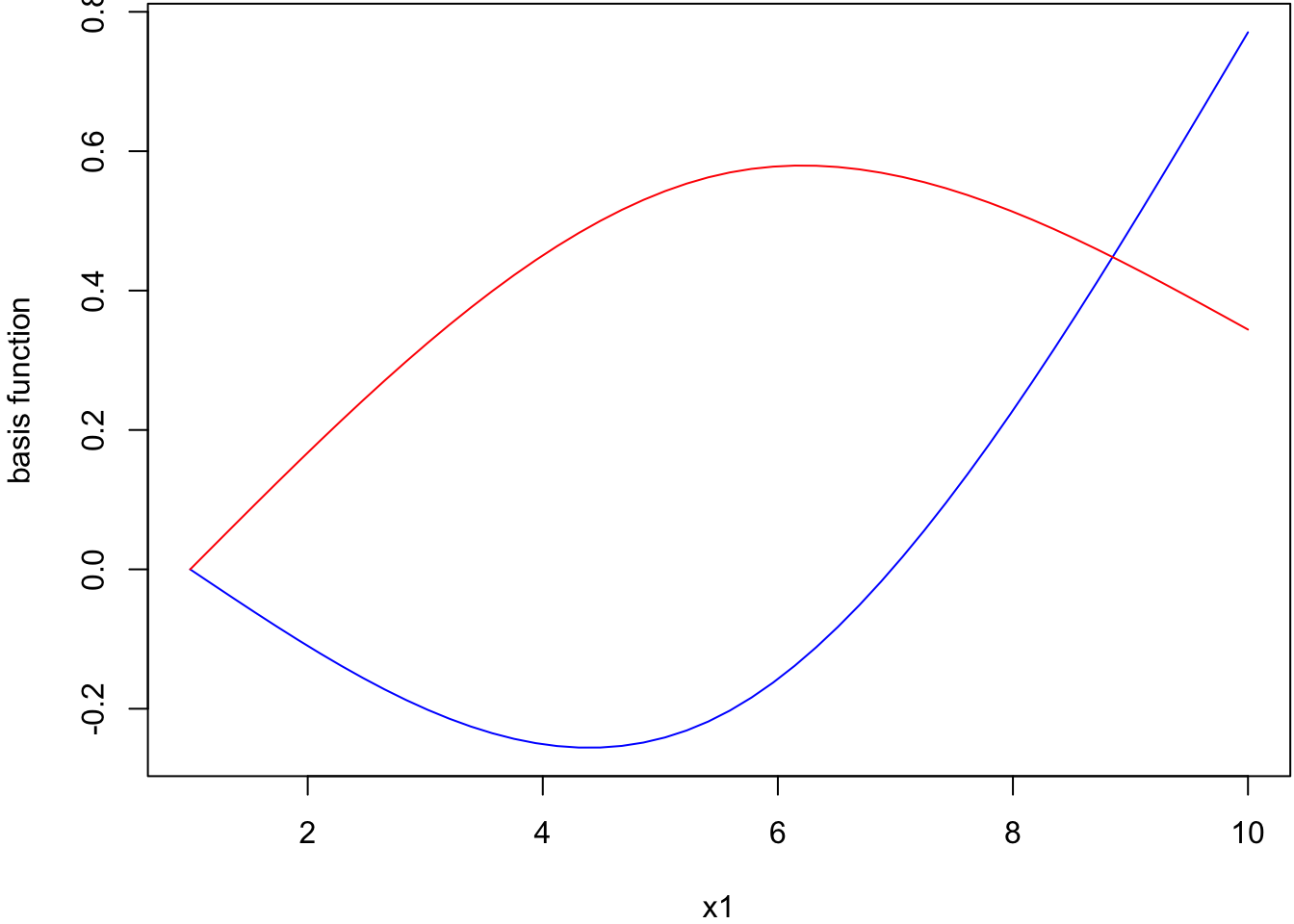> summary(myglm) Call: glm(formula = y ~ ns(x1, df = 2) + x2, family = binomial(), data = df) Deviance Residuals: Min 1Q Median 3Q Max -2.0214 -0.3730 -0.0162 0.5762 1.7616 Coefficients: Estimate Std. Error z value Pr(>|z|) (Intercept) -10.9229 5.3079 -2.058 0.03960 * ns(x1, df = 2)1 21.3848 10.1318 2.111 0.03480 * ns(x1, df = 2)2 6.3266 2.1103 2.998 0.00272 ** x2 0.7342 0.6089 1.206 0.22795 --- Signif. codes: 0 '***' 0.001 '**' 0.01 '*' 0.05 '.' 0.1 ' ' 1 (Dispersion parameter for binomial family taken to be 1) Null deviance: 68.029 on 49 degrees of freedom Residual deviance: 35.682 on 46 degrees of freedom AIC: 43.682 Number of Fisher Scoring iterations: 7 > anova(myglm, test="LRT") Analysis of Deviance Table Model: binomial, link: logit Response: y Terms added sequentially (first to last) Df Deviance Resid. Df Resid. Dev Pr(>Chi) NULL 49 68.029 ns(x1, df = 2) 2 30.755 47 37.274 2.097e-07 *** x2 1 1.592 46 35.682 0.207 --- Signif. codes: 0 '***' 0.001 '**' 0.01 '*' 0.05 '.' 0.1 ' ' 1 True probabilities vs. estimated probabilities. > plot(p, myglm$fitted.values, pch=19); abline(0,1)